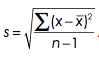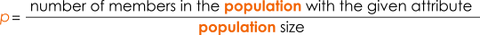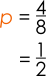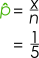# Further Mathematics - Exam Revision

## Population, samples and simple random sampling

Authored By Carmen Popescu-Rose 0 Comment(s)

# POPULATIONS AND SAMPLES

A population consists of all members (people, objects or events) of a specified group about which information is sought.

Examples

• all the teenagers in a country;
• all the students in a school;
• all the kangaroos in Australia.
•

A sample is a representative subset of the population and it consists of some members (people, objects or events) of the population that we collect to make inferences.

Examples

• a group of teenagers in a country;
• a group of students in a school;
• a group of kangaroos in Australia.
•

If the sample is random and large enough, the information collected from the sample can be used to make inferences about the population.Note: Populations consist of very large numbers of members; the example with the cats above is used for visualisation purposes.

 Population parameters Sample statistics population mean = μ standard deviation = σ population size = N (large size) population proportion = p sample mean = x̄ standard deviation = ssample size = n (manageable size) sample proportion = p̂ * hard and costly to collect the data * easy and cost effective to collect the data

Population proportion, p, and Sample proportion

The population proportion is constant; it's value does not change.PopulationIf the group of children shown above represents the population of children in a tutoring class, for example, then the proportion of girls iswhere X is the number of girls in the population and N is the population size.The sample proportion is variable; it's value changes from sample to sample.In the table below there are some examples of sample proportions corresponding to the size of the sample.

 Sample Sample proportion, p̂x = 3 girls n = 5 childrenx = 1 girl n = 5 childrenSimple random sampling

A simple random sample is a subset of a population with the following properties:

* every member of the population is chosen entirely by chance;

every member of the population has an equal chance of being selected;

* all samples have the same size, n;

* every possible sample of size n has an equal chance of being selected.

Methods

Simple random sampling has the advantage that is easy to perform. It works well for a small sample size.

Simple random sampling can be done using

* the lottery type of selection. Each number is written an a ball and put in a lottery machine and selected one by one.

* the old "pick a number from a hat/container/box"* a random number generator which can be a calculator, a software or an online number generator.